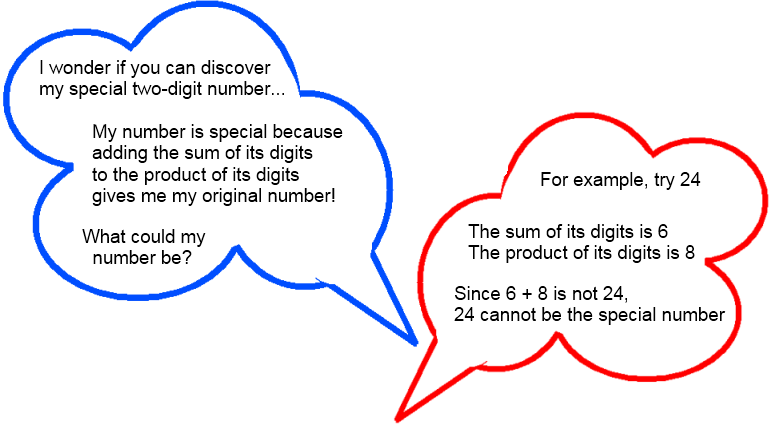### Submit a Resource

NRICH: Special Numbers

One day our teacher asked us a puzzling question:Can you find a special number?
Can you find more than one?
Can you find them all?

One way to convince yourself that you’ve found all the special numbers is to use a little algebra…
Any two-digit number can be represented algebraically as 10a+b.
a is the tens digit and b is the units digit.

Write down the algebraic expressions for the sum and the product of the digits.
Now write an equation that all special numbers will satisfy. See the hint if you’re not sure how to do this.
Solve the equation, and use it to convince yourself that you have found all the special numbers.

There are other sorts of special two-digit numbers…

• I add twice the tens digit to the units digit, then add this to the product of the digits. I get back to my original number.
• I add three times the tens digit to the units digit, then add this to the product of the digits. I get back to my original number.
• I add four times… or five times… or…
Can you use algebra to help you to find these special numbers?
##### Age 11 to 14

Math Topics
Algebra & Pre-Algebra, Linear Equations, Quadratic Equations
Middle School, Educator
Descriptions of PDFs

## Organization

NRICH (University of Cambridge)

Challenge
PDF File